# What Is R Squared And Negative R Squared

## R Squared – A Way Of Evaluating Regression

Regression is a way of fitting a function to a set of data.  For instance, maybe you have been using satellites to count the number of cars in the parking lot of a bunch of Walmart stores for the past couple of years.  You also know the quarterly sales that Walmart had during that time frame from their earnings report.  You want to find a function that relates the two, so that you can use your satellites to count the number of cars and predict Walmart’s quarterly earnings.  (In order to get an advantage in the stock market)

In order to generate that function you would use regression analysis.  But after you generated the car to profit relationship function how can you tell if it is a good quality?  After all, if you are using it to try to predict the stock market, you will be betting real money on it.  You need to know, is your model a good fit?  A bad fit?  Mediocre?  One commonly used metric for determining goodness of fit is R squared, or more formally, the coefficient of determination.

This post goes over R2, and by the end you will understand what it is and how to calculate it, but unfortunately you won’t have a good rule of thumb for what R2 value is good enough for your analysis, because it is entirely problem dependent.  Any R squared value greater than zero means that the regression analysis did better than just using a horizontal line through the mean value.  In the rare cases you get a negative r squared value, you should probably rethink your regression analysis, especially if you are forcing an intercept.

## What Is R Squared?

We will get into the equation for R2 in a little bit, but first what is R squared?   It is how much better your regression line is than a simple horizontal line through the mean of the data.  In the plot below the blue line is the data that we are trying to generate a regression to and the horizontal red line is the average of that data.The red line is the value that gives the lowest summed squared error to the blue data points, assuming you had no other information about the blue data points other than their y value.  This is shown in the plot below.  For the blue data points, only the y values are available.  You don’t know anything else about those values.   You can download the Excel file I used to generate these plots and tables here.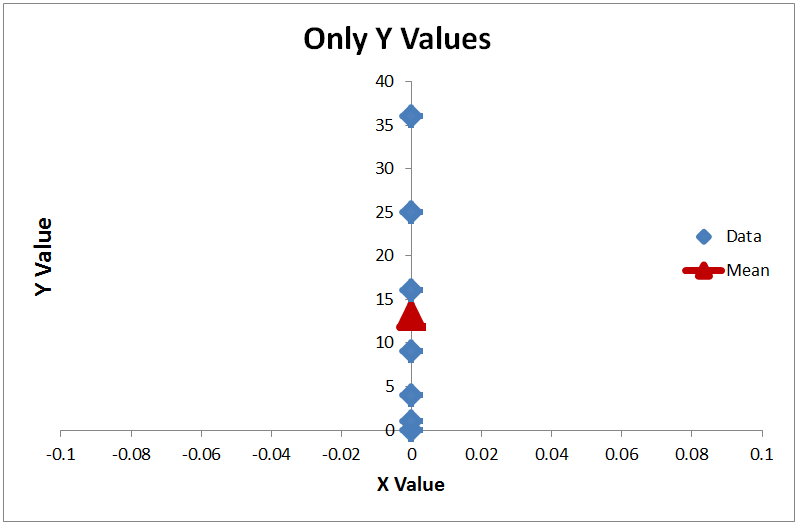If you want to select a value that gives you the lowest summed squared error, the value that you would select was the mean value, shown as the red triangle.  A different way to think about that assertion is this.  If I took all 7 of the y points (0, 1, 4, 9, 16, 25, 36), rearranged them into a random order, and made you guess the value of all 7 points before revealing any of their order, what strategy would give you the minimum sum squared error?   That strategy is to guess the mean value for all the points.

With regression the question is, now that you have more information (the X values in this case) can you make a better approximation than just guessing the mean value?  And the R squared value answers the question, how much better did you do?

That is actually a pretty intuitive understanding.  First calculate how much error you would have if you don’t even try to do regression, and instead just guess the mean of all the values.  That is the total error.  It could be low if all the data is clustered together, or it could be high if the data is spread out.

SS stands for summed squared error, which is how the error is calculated.  To get the total sum squared error you

• For every data point subtract that mean value from the data point value
• Square that difference

Add up all of the squares.  This results in summed squared error## Get The Regression Error

Next calculate the error in your regression values against the true values.  This is your regression error.  Ideally the regression error is very low, near zero.The ratio of the regression error against the total error tells you how much of the total error remains in your regression model.  Subtracting that ratio from 1.0 gives how much error you removed using the regression analysis.  That is R2

In actual equation form it isTo get the total error, you subtract the mean value from each data point, and square the results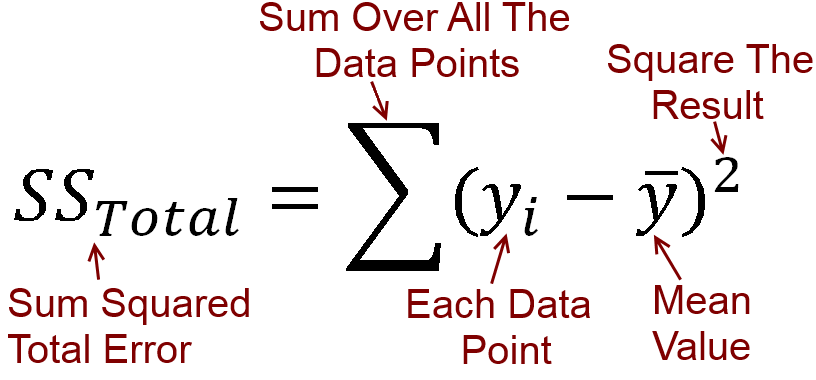For the sum squared regression error, the equation is the same except you use the regression prediction instead of the mean value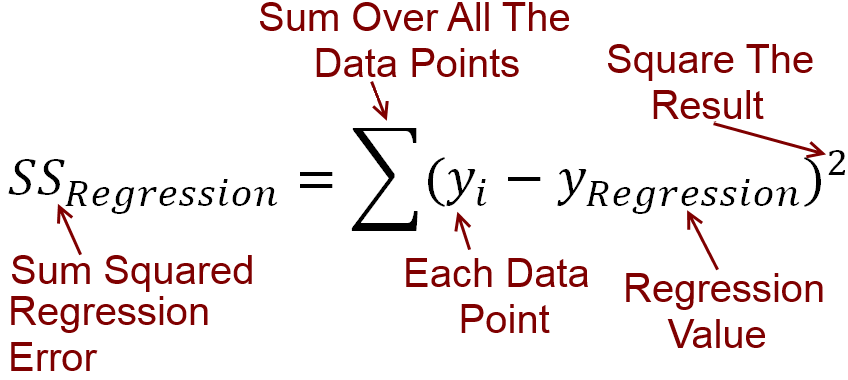## What is a Good R Squared Value?

In most statistics books, you will see that an R squared value is always between 0 and 1, and that the best value is 1.0.   That is only partially true. The lower the error in your regression analysis relative to total error, the higher the R2 value will be.  The best R2 value is 1.0.  To get that value you have to have zero error in your regression analysis.However R2 is not truly limited to a lower bound of zero.  You can get a negative r squared value.

## What Does A Negative R Squared Value Mean?

For practical purposes, the lowest R2 you can get is zero, but only because the assumption is that if your regression line is not better than using the mean, then you will just use the mean value.  However if your regression line is worse than using the mean value, the r squared value that you calculate will be negative.

For instance.  Let’s say that you wanted to make a prediction on the population of one of the states in the United States.   I am not giving you any information other than the population of all 50 states, based on the 2010 census.  I.e.  I am not telling you the name of the state you are trying to make the prediction on, you just have to guess the population (in millions) of all the states in a random order.   The best you could do here is take the mean value.  Your total squared error would be 2298.2

If you used something other than mean, for instance took the median, the summed squared error would be 2247.2

Which when converted into R2 is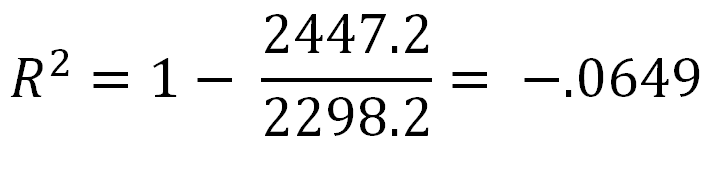And you get a negative R2 number

## Another Way To Get A Negative R Squared Value

The most common way to end up with a negative r squared value is to force your regression line through a specific point, typically by setting the intercept.  The way the ordinary least squares regression equations work is by making a line that passes through a specified point and has the lowest possible sum squared error while still passing through the specified point.

If the point that is chosen is the mean value of x and y, the resulting line will have the lowest possible sum squared error, and the highest possible R-squared value.  If you chose the mean x and y you cannot get a negative r squared value.

However if you specify a different point for the regression line to go through, you will still get the line that generates the lowest sum squared error through that point, but that doesn’t mean that line is good.  For instance in this chart from ExcelThe intercept for both of the regression lines was set at zero.  For the regression line for the blue points, that is not too far off of the best possible regression line, and so the resulting R2 value is positive.  However for the regression line for the red points, the true intercept should be around 120, so setting the intercept to be 0 forces the regression line far away from where it should be.  The result is that the regression sum squared error is greater than if you used used the mean value, and hence a negative r squared value is the result.

The assertion that the R squared value has to be greater than or equal to zero is based on the assumption that if you get a negative R squared value, you will dump whatever regression calculation you are using and just go with the mean value.

The take away for R2 is

• An R2 of 1.0 is the best. It means you have no error in your regression.
• An R2 of 0 means your regression is no better than taking the mean value, i.e. you are not using any information from the other variables
• A Negative R2 means you are doing worse than the mean value. However maybe summed squared error isn’t the metric that matters most to you and this is OK.  (For instance, maybe you care most about mean absolute error instead)

## R Squared Example

As an example of how to calculate R squared, let’s look at this data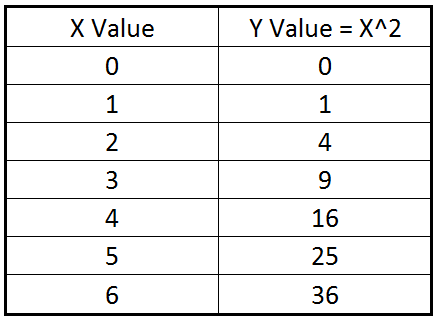This data is just the numbers 0 through 6, with the y value being the square of those numbers.  The linear regression equation for this data is

y = 6x-5

and is plotted on the graph below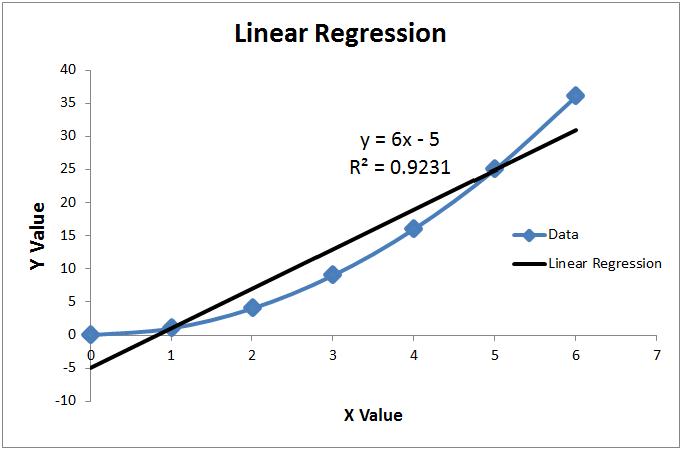Excel has calculated the R2 of this equation to be .9231.  How can we duplicate that manually?

Well the equation isSo we need to find the total summed squared error (based off the mean) and the summed squared error based off the regression line.

The mean value of the y values of the data (0, 1, 4, 9, 16, 25, 36) is 13To find the total summed square error, we will subtract 13 from each of the y values, square that result, and add up all of the squares.  Graphically, this is shown below.  At every data point, the distance between the red line and the blue line is squared, and then all of those squares are summed upThe total sum squared error is 1092, with most of the error coming from the edges of the chart where the mean is the farthest way from the true valueNow we need find the values that our regression line of y = 6x-5 predicts, and get the summed squared error of that.  For the sum squared value, we will subtract each y regression value from the true value, take the square, and sum up all of the squares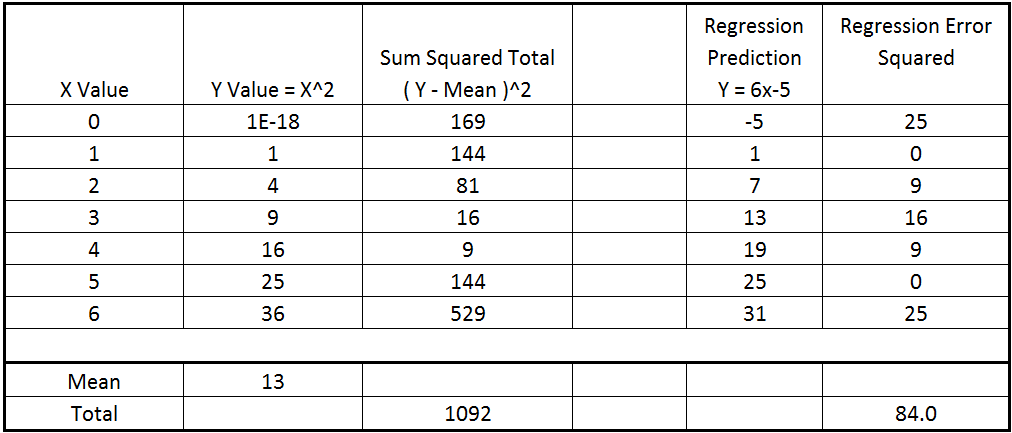So the total summed squared error of the linear regression is 84, and the total summed squared error is 1092 based on the mean value.

Plugging these numbers into the R2 equation we getWhich is the same value that Excel calculated.

A different way to think about the same result would be that we have 84/1092 = 7.69 % of the total error remaining.  Basically, if someone had just given us the y values, and then told us that they were going to pick random order those y values and we had to guess what they all were, the best guess we could have made was the mean for each one.   But if now they give us the X value, and tell us to try to guess the Y value, we can use the linear regression line and remove 92.31% of the error from our guess.

But Wait, Can’t We Do Better?

We just showed a linear regression line that produced an R squared value of .9231 and said that that was the best linear fit we could make based on the summed squared error metric.  But couldn’t we do better with a different regression fit?

Well the answer is yes, of course we could.  We used a linear regression, i.e. a straight line, on this data.  However the data itself wasn’t linear.  Y is the square of x with this data.  So if we used a square regression, and in fact just used the equation y = x2, we get a much better fit, which is shown below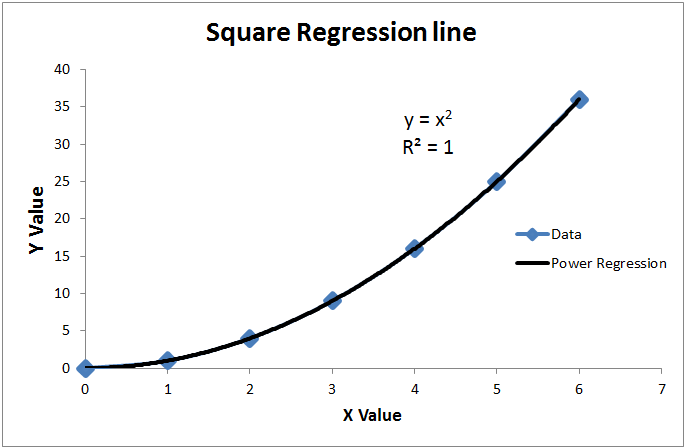Here we have an R2 of 1.0, because the regression line exactly matches the data, and there is no remaining error.   However the fact that we were able to do this is somewhat beside the point for this R2 explanation.  We were able to find an exact match for this data only because it is a toy data set.  The Y values were built as the square of the X values, so it is no surprise that making a regression that utilized that fact gave a good match.

For most data sets, an exact match will not be able to be generated because the data will be noisy and not a simple equation.  For instance, an economist might be doing a study to determine what attributes of a person correlate to their adult profession and income.  Some of those attributes could be height, childhood interests, parent’s incomes, school grades, SAT scores etc.   It is unlikely that any of those will have a perfect R2 value, in fact the R squared value some of them might be quite low.  But there are times that even a low R2 value could be of interest

Any R2 value above 0.0 indicates that there could be some correlation between the variable and the result, although very low values are likely just random noise.

## An Odd Special Case For R Squared

Just for fun, what do you think the R2 of this linear regression line for this data is?Here we have a pure horizontal line, all the data is 5.0.

As it turns out, the R squared value of a linear regression on this data is undefined.   Excel will display the value as N/A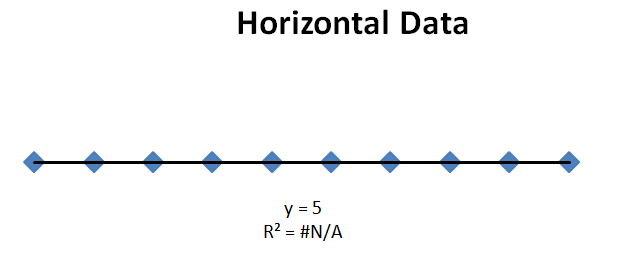What has happened in this example is that the total summed squared error is equal to zero.   All the data values exactly equal the mean value.  So there is zero error if you just estimate the mean value.

Of course, there is also zero error for the regression line.  You end up with a zero divided by zero term in the R squared equation, which is undefined.# Binomial Equation – Made Easy To Remember

The binomial equation calculates the probability of getting a certain outcome after a number of discrete events.  For instance, if your team has a 60% chance of winning any single game against another team, what are the odds that they will win at least 4 out of 7 games in the championship series against that other team?

The most common equation you see for the binomial distribution is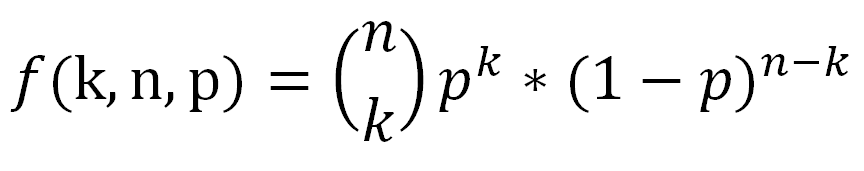As far as what the letters mean

• f – just means it is a function of k, n, p
• k – This is the number of successful events. e. the total number of heads in the coin flips, or the total number of outcome A out of A/B
• n – This is the total number of events. This would be all the flips, regardless of the outcome
• p – This is a decimal number between 0 and 1 inclusive representing the probability of a successful event on a single trial. For instance if you were rolling a die and it only counted if you got a 6, then p would be 1/6 = .1667

However I think the equation is easier to remember when written in a slightly different way, as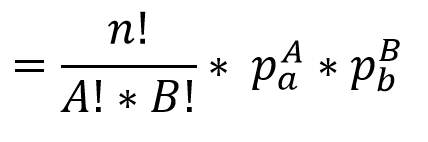Here A is the number of times outcome A occurs, so it is equal to k from the previous equation.  B is the number of times outcome B occurs, and is equal to (n-k) when there are two events.  pa is the probability that outcome A occurs and pb is the probability that outcome B occurs

## What You Already Know In The Binomial Equation

The reason I like the equation in this form is that it builds on information that you already know.  For instance, say that you have an outcome that has a 1/6 chance of occurring, like rolling a 6 on a die.  What are the odds that event will occur 10 times in a row?   Well the odds of that happening are 1/6 raised to the 10th power.  You likely already know that, and in equation form it would beNow let’s say that you have two different outcomes, A and B.   What is the probability that outcome A will occur A times in a row, and then outcome B will occur B times in a row?   This is just the probability of A, raised to the power of A times, multiplied by the probability of B, raised to the power of B times.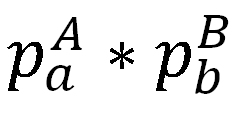For instance, if you wanted to calculate the odds of rolling a die 5 times in a row and getting 6 every time, and then flipping a coin 4 times in a row and getting heads every time it would just be  1/6 raised to the 5th power, multiplied by 1/2 raised to the 4th power.  That is simple enough, easy to understand, and frankly you don’t need a special equation in order to remember it.  And that is the second half of the binomial equation.The second half of the binomial equation is just   “If outcome A had to occur all the times in a row, then outcome B all the times in a row, what is the probability that string of outcomes would occur?”

## 1st Half Of Binomial Equation

Ok, that is the second half of the equation with the exponentials, what about the first half of the equation?This is the combination equation.  There are a lot of interesting things to know about the combination equation, and those are discussed in this blog post on combinations and permutations.  For our purposes right now, the best way to think of this part of the equation is as such:   It is the number of different orders of ways you can do outcome A and outcome B.   i.e. it is the number of different tries you get at making your desired outcome happen.

As an example, let’s say that outcome A was rolling a 6 on a die, and outcome B was flipping a heads on a coin, and you needed to do that two times each.   If you had to roll a 6 twice in a row, and flip the coin twice in a row the probability would beAs we showed before.   However now let me tell you that you get to try again, however many times you can reorder the events.  For instance, the first trial was

• Roll-Roll-Flip-Flip

So that is one order.  But you can also try again

• Roll-Flip-Roll-Flip

And

• Roll-Flip-Flip-Roll

And so on.  There are in fact 6 unique orderings of 2 rolls and 2 flips.   This can be calculated by 4! / (2!*2!)  =   24 / (2*2) = 6       here the 4! Is because the total number of events is 4  (2+2)   and each of the two factorial is because there are two trials of event A, and two trials of event B.

The 6 unique orders of rolls and flips, as A’s and B’s are

• A-A-B-B
• A-B-A-B
• A-B-B-A
• B-A-B-A
• B-B-A-A
• B-A-A-B

So the first half of the equationTells you the number of times you get to try to get a specific outcome, and the second half of the equationTells you the probability of that outcome if done a single time.   Combined they give the full probability of an outcome## Example Problem Solved

Let’s take it back to the starting example.  Your team has a 60% chance of winning.  What are the odds they will win exactly 4 games in 7 ?

• Outcome A is that your team wins. This occurs 4 times, and a has a probability of .6
• Outcome B is that the other team wins. This occurs 3 times and has a probability of .4.

There are 7 events in all.  The equation becomesSo the odds of your team winning exactly 4 games are 29.03%.

## What Are The Odds Of At Least 4 Games?

But wait, the real question we asked was the odds of winning at least 4 games, not exactly 4 games.  Your team could win 5 games, or 6 games, or all 7.  That means we have to calculate the answer again for each of those possible number of wins.  That calculation is done belowThe end result is that if your team has a 60% chance of winning any single game, you expect that they have a 71.02% chance of winning at least 4 games in a 7 game series.

If you increased the number of games, and went best 5 of 9, or best 6 of 11 the likelihood of the team with the higher winning percentage winning the series increases.

## Multinomial Equation

There is another reason why I like the binomial equation rewritten the way we did it.  That is because it is easy to expand that equation from a Binomial equation to a Multinomial equation.  I.e. it is easy to change from 2 outcomes to 3 or more outcomes.

What would be a multinomial event?  Well for instance, instead of having two teams play, think of three people racing in a track meet.  So instead of calculating the odds of one team winning 4 games out of 7, what are the odds that person A will win 4 races out of 7, person B will win 2 races out of 7, and person C will win one?

Here assume the odds of person A, B, and C winning any given race are 50%, 40%, and 10% respectively.

Getting the equation for 3 possible outcomes from the 2 possible outcome equation is almost trivial.  It is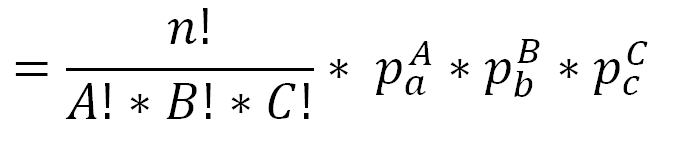Once again, the second half of the equation is the probability that that specific chain of outcomes will occur, and the first half of the equation is the number of different paths you can take to get there.

For this specific racing problem the equation becomes